## The Finite Element Method (FEM)

General   Finite Element Method

### An Introduction to the Finite Element Method

The description of the laws of physics for space- and time-dependent problems are usually expressed in terms of partial differential equations (PDEs). For the vast majority of geometries and problems, these PDEs cannot be solved with analytical methods. Instead, an approximation of the equations can be constructed, typically based upon different types of discretizations. These discretization methods approximate the PDEs with numerical model equations, which can be solved using numerical methods. The solution to the numerical model equations are, in turn, an approximation of the real solution to the PDEs. The finite element method (FEM) is used to compute such approximations.

Take, for example, a function u that may be the dependent variable in a PDE (i.e., temperature, electric potential, pressure, etc.) The function u can be approximated by a function uh using linear combinations of basis functions according to the following expressions:

(1)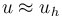and

(2)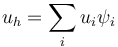Here, ψi denotes the basis functions and ui denotes the coefficients of the functions that approximate u with uh. The figure below illustrates this principle for a 1D problem. u could, for instance, represent the temperature along the length (x) of a rod that is nonuniformly heated. Here, the linear basis functions have a value of 1 at their respective nodes and 0 at other nodes. In this case, there are seven elements along the portion of the x-axis, where the function u is defined (i.e., the length of the rod).The function u (solid blue line) is approximated with uh (dashed red line), which is a linear combination of linear basis functions (ψi is represented by the solid black lines). The coefficients are denoted by u0 through u7.

The function u (solid blue line) is approximated with uh (dashed red line), which is a linear combination of linear basis functions (ψi is represented by the solid black lines). The coefficients are denoted by u0 through u7.

One of the benefits of using the finite element method is that it offers great freedom in the selection of discretization, both in the elements that may be used to discretize space and the basis functions. In the figure above, for example, the elements are uniformly distributed over the x-axis, although this does not have to be the case. Smaller elements in a region where the gradient of u is large could also have been applied, as highlighted below.The function u (solid blue line) is approximated with uh (dashed red line), which is a linear combination of linear basis functions (ψi is represented by the solid black lines). The coefficients are denoted by u0 through u7.

The function u (solid blue line) is approximated with uh (dashed red line), which is a linear combination of linear basis functions (ψi is represented by the solid black lines). The coefficients are denoted by u0 through u7.

Both of these figures show that the selected linear basis functions include very limited support (nonzero only over a narrow interval) and overlap along the x-axis. Depending on the problem at hand, other functions may be chosen instead of linear functions.

Another benefit of the finite element method is that the theory is well developed. The reason for this is the close relationship between the numerical formulation and the weak formulation of the PDE problem (see the section below). For instance, the theory provides useful error estimates, or bounds for the error, when the numerical model equations are solved on a computer.

Looking back at the history of FEM, the usefulness of the method was first recognized at the start of the 1940s by Richard Courant, a German-American mathematician. While Courant recognized its application to a range of problems, it took several decades before the approach was applied generally in fields outside of structural mechanics, becoming what it is today.Finite element discretization, stresses, and deformations of a wheel rim in a structural analysis. Finite element discretization, stresses, and deformations of a wheel rim in a structural analysis.

### Algebraic Equations, Ordinary Differential Equations, Partial Differential Equations, and the Laws of Physics

The laws of physics are often expressed in the language of mathematics. For example, conservation laws such as the law of conservation of energy, conservation of mass, and conservation of momentum can all be expressed as partial differential equations (PDEs). Constitutive relations may also be used to express these laws in terms of variables like temperature, density, velocity, electric potential, and other dependent variables.

Differential equations include expressions that determine a small change in a dependent variable with respect to a change in an independent variable (x, y, z, t). This small change is also referred to as the derivative of the dependent variable with respect to the independent variable.

Say there is a solid with time-varying temperature but negligible variations in space. In this case, the equation for conservation of internal (thermal) energy may result in an equation for the change of temperature, with a very small change in time, due to a heat source g:

(3)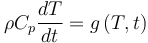Here,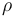denotes the density and Cp denotes the heat capacity. Temperature, T, is the dependent variable and time, t, is the independent variable. The function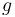may describe a heat source that varies with temperature and time. Eq. (3) states that if there is a change in temperature in time, then this has to be balanced (or caused) by the heat source. The equation is a differential equation expressed in terms of the derivatives of one independent variable (t). Such differential equations are known as ordinary differential equations (ODEs).

In some situations, knowing the temperature at a time t0, called an initial condition, allows for an analytical solution of Eq. (3) that is expressed as:

(4)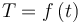The temperature in the solid is therefore expressed through an algebraic equation (4), where giving a value of time, t1, returns the value of the temperature, T1, at that time.

Oftentimes, there are variations in time and space. The temperature in the solid at the positions closer to a heat source may, for instance, be slightly higher than elsewhere. Such variations further give rise to a heat flux between the different parts within the solid. In such cases, the conservation of energy can result in a heat transfer equation that expresses the changes in both time and spatial variables (x), such as:

(5)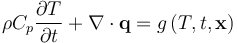As before, T is the dependent variable, while x (x = (x, y, z)) and t are the independent variables. The heat flux vector in the solid is denoted by q = (qx, qy, qz) while the divergence of q describes the change in heat flux along the spatial coordinates. For a Cartesian coordinate system, the divergence of q is defined as:

(6)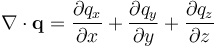Eq. (5) thus states that if there is a change in net flux when changes are added in all directions so that the divergence (sum of the changes) of q is not zero, then this has to be balanced (or caused) by a heat source and/or a change in temperature in time (accumulation of thermal energy).

The heat flux in a solid can be described by the constitutive relation for heat flux by conduction, also referred to as Fourier’s law:

(7)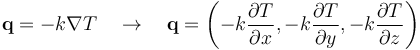In the above equation, k denotes the thermal conductivity. Eq. (7) states that the heat flux is proportional to the gradient in temperature, with the thermal conductivity as proportionality constant. Eq. (7) in (5) gives the following differential equation:

(8)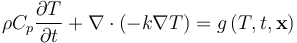Here, the derivatives are expressed in terms of t, x, y, and z. When a differential equation is expressed in terms of the derivatives of more than one independent variable, it is referred to as a partial differential equation (PDE), since each derivative may represent a change in one direction out of several possible directions. Further note that the derivatives in ODEs are expressed using d, while partial derivatives are expressed using the more curly .

In addition to Eq. (8), the temperature at a time t0 and temperature or heat flux at some position x0 could be known as well. Such knowledge can be applied in the initial condition and boundary conditions for Eq. (8). In many situations, PDEs cannot be resolved with analytical methods to give the value of the dependent variables at different times and positions. It may, for example, be very difficult or impossible to obtain an analytic expression such as:

(9)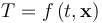from Eq. (8).

Rather than solving PDEs analytically, an alternative option is to search for approximate numerical solutions to solve the numerical model equations. The finite element method is exactly this type of method – a numerical method for the solution of PDEs.

Similar to the thermal energy conservation referenced above, it is possible to derive the equations for the conservation of momentum and mass that form the basis for fluid dynamics. Further, the equations for electromagnetic fields and fluxes can be derived for space- and time-dependent problems, forming systems of PDEs.

Continuing this discussion, let's see how the so-called weak formulation can be derived from the PDEs.

### The Finite Element Method from the Weak Formulation: Basis Functions and Test Functions

Assume that the temperature distribution in a heat sink is being studied, given by Eq. (8), but now at steady state, meaning that the time derivative of the temperature field is zero in Eq. (8). The domain equation for the model domain, Ω, is the following:

(10)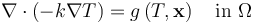Further, assume that the temperature along a boundary (Ω1) is known, in addition to the expression for the heat flux normal to some other boundaries (Ω2). On the remaining boundaries, the heat flux is zero in the outward direction (Ω3). The boundary conditions at these boundaries then become:

(11)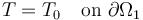(12)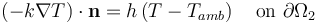(13)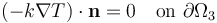where h denotes the heat transfer coefficient and Tamb denotes the ambient temperature. The outward unit normal vector to the boundary surface is denoted by n. Equations (10) to (13) describe the mathematical model for the heat sink, as shown below.The domain equation and boundary conditions for a mathematical model of a heat sink.

The domain equation and boundary conditions for a mathematical model of a heat sink.

The next step is to multiply both sides of Eq. (10) by a test function φ and integrate over the domain Ω:

(14)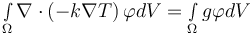The test function φ and the solution T are assumed to belong to Hilbert spaces. A Hilbert space is an infinite-dimensional function space with functions of specific properties. It can be viewed as a collection of functions with certain nice properties, such that these functions can be conveniently manipulated in the same way as ordinary vectors in a vector space. For example, you can form linear combinations of functions in this collection (the functions have a well-defined length referred to as norm) and you can measure the angle between the functions, just like Euclidean vectors.

Indeed, after applying the finite element method on these functions, they are simply converted to ordinary vectors. The finite element method is a systematic way to convert the functions in an infinite dimensional function space to first functions in a finite dimensional function space and then finally ordinary vectors (in a vector space) that are tractable with numerical methods.

The weak formulation is obtained by requiring (14) to hold for all test functions in the test function space instead of Eq. (10) for all points in Ω. A problem formulation based on Eq. (10) is thus sometimes referred to as the pointwise formulation. In the so-called Galerkin method, it is assumed that the solution T belongs to the same Hilbert space as the test functions. This is usually written as φ ϵ H and T ϵ H, where H denotes the Hilbert space. Using Green’s first identity (essentially integration by parts), the following equation can be derived from (14):

(15)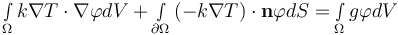The weak formulation, or variational formulation, of Eq. (10) is obtained by requiring this equality to hold for all test functions in the Hilbert space. It is called “weak” because it relaxes the requirement (10), where all the terms of the PDE must be well defined in all points. The relations in (14) and (15) instead only require equality in an integral sense. For example, a discontinuity of a first derivative for the solution is perfectly allowed by the weak formulation since it does not hinder integration. It does, however, introduce a distribution for the second derivative that is not a function in the ordinary sense. As such, the requirement (10) does not make sense at the point of the discontinuity.

A distribution can sometimes be integrated, making (14) well defined. It is possible to show that the weak formulation, together with boundary conditions (11) through (13), is directly related to the solution from the pointwise formulation. And, for cases where the solution is differentiable enough (i.e., when second derivatives are well defined), these solutions are the same. The formulations are equivalent, since deriving (15) from (10) relies on Green’s first identity, which only holds if T has continuous second derivatives.

This is the first step in the finite element formulation. With the weak formulation, it is possible to discretize the mathematical model equations to obtain the numerical model equations. The Galerkin method – one of the many possible finite element method formulations – can be used for discretization.

First, the discretization implies looking for an approximate solution to Eq. (15) in a finite-dimensional subspace to the Hilbert space H so that T ≈ Th. This implies that the approximate solution is expressed as a linear combination of a set of basis functions ψi that belong to the subspace:

(16)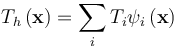The discretized version of Eq. (15) for every test function ψj therefore becomes:

(17)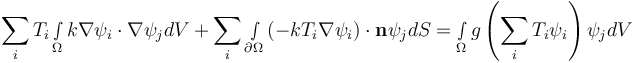The unknowns here are the coefficients Ti in the approximation of the function T(x). Eq. (17) then forms a system of equations of the same dimension as the finite-dimensional function space. If n number of test functions ψj are used so that j goes from 1 to n, a system of n number of equations is obtained according to (17). From Eq. (16), there are also n unknown coefficients (Ti).Finite element discretization of the heat sink model from the earlier figure. Finite element discretization of the heat sink model from the earlier figure.

Once the system is discretized and the boundary conditions are imposed, a system of equations is obtained according to the following expression:

(18)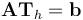where T is the vector of unknowns, T h = {T1, .., Ti, …, Tn}, and A is an nxn matrix containing the coefficients of Ti in each equation j within its components Aji. The right-hand side is a vector of the dimension 1 to n. A is the system matrix, often referred to as the (eliminated) stiffness matrix, harkening back to the finite element method’s first application as well as its use in structural mechanics.

If the source function is nonlinear with respect to temperature or if the heat transfer coefficient depends on temperature, then the equation system is also nonlinear and the vector b becomes a nonlinear function of the unknown coefficients Ti.

One of the benefits of the finite element method is its ability to select test and basis functions. It is possible to select test and basis functions that are supported over a very small geometrical region. This implies that the integrals in Eq. (17) are zero everywhere, except in very limited regions where the functions ψj and ψi overlap, as all of the above integrals include products of the functions or gradients of the functions i and j. The support of the test and basis functions is difficult to depict in 3D, but the 2D analogy can be visualized.

Assume that there is a 2D geometrical domain and that linear functions of x and y are selected, each with a value of 1 at a point i, but zero at other points k. The next step is to discretize the 2D domain using triangles and depict how two basis functions (test or shape functions) could appear for two neighboring nodes i and j in a triangular mesh.Tent-shaped linear basis functions that have a value of 1 at the corresponding node and zero on all other nodes. Two base functions that share an element have a basis function overlap. Tent-shaped linear basis functions that have a value of 1 at the corresponding node and zero on all other nodes. Two base functions that share an element have a basis function overlap.

Two neighboring basis functions share two triangular elements. As such, there is some overlap between the two basis functions, as shown above. Further, note that if i = j, then there is a complete overlap between the functions. These contributions form the coefficients for the unknown vector T that correspond to the diagonal components of the system matrix Ajj.

Say the two basis functions are now a little further apart. These functions do not share elements but they have one element vertex in common. As the figure below indicates, they do not overlap.Two basis functions that share one element vertex but do not overlap in a 2D domain. Two basis functions that share one element vertex but do not overlap in a 2D domain.

When the basis functions overlap, the integrals in Eq. (17) have a nonzero value and the contributions to the system matrix are nonzero. When there is no overlap, the integrals are zero and the contribution to the system matrix is therefore zero as well.

This means that each equation in the system of equations for (17) for the nodes 1 to n only gets a few nonzero terms from neighboring nodes that share the same element. The system matrix A in Eq. (18) becomes sparse, with nonzero terms only for the matrix components that correspond to overlapping ij:s. The solution of the system of algebraic equations gives an approximation of the solution to the PDE. The denser the mesh, the closer the approximate solution gets to the actual solution.The finite element approximation of the temperature field in the heat sink. The finite element approximation of the temperature field in the heat sink.

### Time-Dependent Problems

The thermal energy balance in the heat sink can be further defined for time-dependent cases. The discretized weak formulation for every test function ψj, using the Galerkin method, can then be written as:

(19)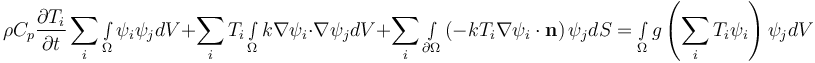Here, the coefficients Ti are time-dependent functions while the basis and test functions depend just on spatial coordinates. Further, the time derivative is not discretized in the time domain.

One approach would be to use FEM for the time domain as well, but this can be rather computationally expensive. Alternatively, an independent discretization of the time domain is often applied using the method of lines. For example, it is possible to use the finite difference method. In its simplest form, this can be expressed with the following difference approximation:

(20)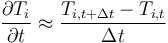Two potential finite difference approximations of the problem in Eq. (19) are given. The first formulation is when the unknown coefficients Ti,t are expressed in terms of t + Δt:

(21)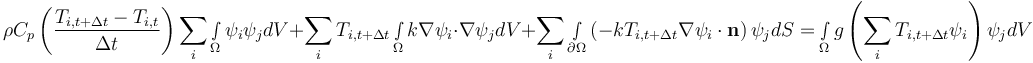If the problem is linear, a linear system of equations needs to be solved for each time step. If the problem is nonlinear, a corresponding nonlinear system of equations must be solved in each time step. The time-marching scheme is referred to as an implicit method, as the solution at t + Δt is implicitly given by Eq. (21).

The second formulation is in terms of the solution at t:

(22)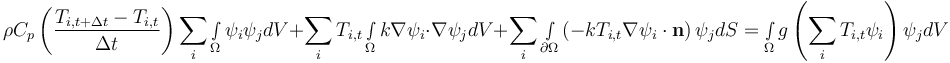This formulation implies that once the solution (Ti,t) is known at a given time, then Eq. (22) explicitly gives the solution at t + Δt (Ti, t+Δt). In other words, for an explicit time-marching scheme, there is no need to solve an equation system at each time step. The drawback with explicit time-marching schemes is that they come with a stability time-stepping restriction. For heat problems, like the case highlighted here, an explicit method requires very small time steps. The implicit schemes allow for larger time steps, cutting the costs for equations like (22) that have to be solved in each time step.

In practice, modern time-stepping algorithms automatically switch between explicit and implicit steps depending on the problem. Further, the difference equation in Eq. (20) is replaced with a polynomial expression that may vary in order and step length depending on the problem and the evolution of the solution with time. A modern time-marching scheme has automatic control of the polynomial order and the step length for the time evolution of the numerical solution.

When it comes to the most common methods that are used, here are a few examples:

• Backwards differentiation formula (BDF) method
• Generalized alpha method
• Different Runge-Kutta methods

### Different Elements

As mentioned above, the Galerkin method utilizes the same set of functions for the basis functions and the test functions. Yet, even for this method, there are many ways (infinitely many, in theory) of defining the basis functions (i.e., the elements in a Galerkin finite element formulation). Let's review some of the most common elements.

For linear functions in 2D and 3D, the most common elements are illustrated in the figure below. The linear basis functions, as defined in a triangular mesh that forms triangular linear elements, are depicted in this figure and this figure above. The basis functions are expressed as functions of the positions of the nodes (x and y in 2D and x, y, and z in 3D).

In 2D, rectangular elements are often applied to structural mechanics analyses. They can also be used for boundary layer meshing in CFD and heat transfer modeling. Their 3D analogy is known as the hexahedral elements, and they are commonly applied to structural mechanics and boundary layer meshing as well. In the transition from hexahedral boundary layer elements to tetrahedral elements, pyramidal elements are usually placed on top of the boundary layer elements.Node placement and geometry for 2D and 3D linear elements. Node placement and geometry for 2D and 3D linear elements.

The corresponding second-order elements (quadratic elements) are shown in the figure below. Here, the edges and surfaces facing a domain boundary are frequently curved, while the edges and surfaces facing the internal portion of the domain are lines or flat surfaces. Note, however, that there is also the option to define all edges and surfaces as curved. Lagrangian and serendipity elements are the most common element types in 2D and 3D. The Lagrangian elements use all of the nodes below (black, white, and gray), while the serendipity elements omit the gray nodes.Second-order elements. If the gray nodes are removed, we get the corresponding serendipity elements. The black, white, and gray nodes are all present in the Lagrangian elements. Second-order elements. If the gray nodes are removed, we get the corresponding serendipity elements. The black, white, and gray nodes are all present in the Lagrangian elements.

Beautiful plots of 2D second-order (quadratic) Lagrangian elements can be found in the blog post "Keeping Track of Element Order in Multiphysics Models". It is difficult to depict the basis of the quadratic basis functions in 3D inside the elements above, but color fields can be used to plot function values on the element surfaces.

When discussing FEM, an important element to consider is the error estimate. This is because when an estimated error tolerance is reached, convergence occurs. Note that the discussion here is more general in nature rather than specific to FEM.

The finite element method gives an approximate solution to the mathematical model equations. The difference between the solution to the numerical equations and the exact solution to the mathematical model equations is the error: e = u - uh.

In many cases, the error can be estimated before the numerical equations are solved (i.e., an a priori error estimate). A priori estimates are often used solely to predict the convergence order of the applied finite element method. For instance, if the problem is well posed and the numerical method converges, the norm of the error decreases with the typical element size h according to O(hα), where α denotes the order of convergence. This simply indicates how fast the norm of the error is expected to decrease as the mesh is made denser.

An a priori estimate can only be found for simple problems. Furthermore, the estimates frequently contain different unknown constants, making quantitative predictions impossible. An a posteriori estimate uses the approximate solution, in combination with other approximations to related problems, in order to estimate the norm of the error.

#### Method of Manufactured Solutions

A very simple, but general, method for estimating the error for a numerical method and PDE problem is to modify the problem slightly, as seen in this blog post, so that a predefined analytical expression is the true solution to the modified problem. The advantage here is that there are no assumptions made regarding the numerical method or the underlying mathematical problem. Additionally, since the solution is known, the error can easily be evaluated. By selecting the analytical expression with care, different aspects of the method and problem can be studied.

Let's take a look at an example to clarify this. Assume that there is a numerical method that solves Poisson’s equation on a unit square (Ω) with homogeneous boundary conditions

(23)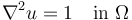(24)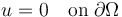This method can be used to solve a modified problem

(25)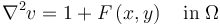(26)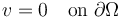where

(27)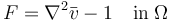Here,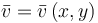is an analytical expression that can be selected freely. If also

(28)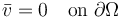then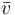is the exact solution to the modified problem and the error can be directly evaluated:

(29)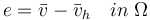The error and its norm can now be computed for different choices of discretization and different choices of. The error to the modified problem can be used to approximate the error for the unmodified problem if the character of the solution to the modified problem resembles the solution to the unmodified problem. In practice, it may be difficult to know if this is the case – a drawback of the technique. The strength of the approach lies in its simplicity and generality. It can be used for nonlinear and time-dependent problems, and it can be used for any numerical method.

#### Goal-Oriented Estimates

If a function, or functional, is selected as an important quantity to estimate from the approximate solution, then analytical methods can be used to derive sharp error estimates, or bounds, for the computational error made for this quantity. Such estimates rely on a posteriori evaluation of a PDE residual and computation of the approximation to a so-called dual problem. The dual problem is directly related to (and defined by) the selected function.

The drawback of this method is that it hinges on the accurate computation of the dual problem and only gives an estimate of the error for the selected function, not for other quantities. Its strength lies in the fact that it is rather generally applicable and the error is computable with reasonable efforts.

#### Mesh Convergence

Mesh convergence is a simple method that compares approximate solutions obtained for different meshes. Ideally, a very fine mesh approximation solution can be taken as an approximation to the actual solution. The error for approximation on the coarser meshes can then be directly evaluated as

(30)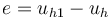In practice, computing an approximation for a very much finer mesh than those of interest can be difficult. Therefore, it is customary to use the finest mesh approximation for this purpose. It is also possible to estimate the convergence from the change in the solution for each mesh refinement. The change in the solution should become smaller for each mesh refinement if the approximate solution is in a converging area, so that it moves closer and closer to the real solution.

The figure below shows a structural mechanics benchmark model for an elliptic membrane where only one fourth of the membrane is treated thanks to symmetry. The load is applied on the outer edge of the geometry while symmetry is assumed at the boundaries along the x- and y-axis.Benchmark model of an elliptic membrane. The load is applied at the outer edge while symmetry is assumed at the edges positioned along the x- and y-axis (roller support).

Benchmark model of an elliptic membrane. The load is applied at the outer edge while symmetry is assumed at the edges positioned along the x- and y-axis (roller support).

The numerical model equations are solved for different mesh types and element sizes. For example, the figure below depicts the rectangular Lagrange elements used for the quadratic basis functions according this previous figure.Rectangular elements used for the quadratic base functions. Rectangular elements used for the quadratic base functions.

The stresses and strains are evaluated in the point according to this earlier figure. The relative values obtained for σx at this point are shown in the chart below. This value should be zero and any deviation from zero is therefore an error. To get a relative error, the computed σx is divided by the computed σy in order to get the correct order of magnitude for a relative error estimate.Relative error in σx at the evaluation point in the previous figure for different elements and element sizes (element size = h). Quad refers to rectangular elements, which can be linear or with quadratic basis functions. Relative error in σx at the evaluation point in the previous figure for different elements and element sizes (element size = h). Quad refers to rectangular elements, which can be linear or with quadratic basis functions.

The above plot shows that the relative error decreases with decreasing element size (h) for all elements. In this case, the convergence curve becomes steeper as the order of the basis functions (elements order) becomes higher. Note, however, that the number of unknowns in the numerical model increases with the element order for a given element size. This means that when we increase the order of the elements, we pay the price for higher accuracy in the form of increased computational time. An alternative to using higher-order elements is therefore to implement a finer mesh for the lower-order elements.

After computing a solution to the numerical equations, uh, a posteriori local error estimates can be used to create a denser mesh where the error is large. A second approximate solution may then be computed using the refined mesh.

The figure below depicts the temperature field around a heated cylinder subject to fluid flow at steady state. The stationary problem is solved twice: once with the basic mesh and once with a refined mesh controlled by the error estimation that is computed from the basic mesh. The refined mesh provides higher accuracy in temperature and heat flux, which may be required in this specific case.Temperature field around a heated cylinder that is subjected to a flow computed without mesh refinement (top) and with mesh refinement (bottom). Temperature field around a heated cylinder that is subjected to a flow computed without mesh refinement (top) and with mesh refinement (bottom).

For convective time-dependent problems, there is also the option to convect the refinement of the mesh via the solution in a previous time step. For instance, in the figure below, the phase field is used to compute the interface between an ink droplet and air in an inkjet. The interface is given by the isosurface of the phase field function, where its value is equal to 0.5. At this interface, the phase field function goes from 1 to 0 very rapidly. We can use the error estimate to automatically refine the mesh around these steep gradients of the phase field function, and the flow field can be used to convect the mesh refinement to use a finer mesh just in front of the phase field isosurface.The mesh refinement follows the train of droplets from an inkjet in a time-dependent, two-phase flow problem. The mesh refinement follows the train of droplets from an inkjet in a time-dependent, two-phase flow problem.

### Additional Finite Element Formulations

In the examples above, we have formulated the discretization of the model equations using the same set of functions for the basis and test functions. One finite element formulation where the test functions are different from the basis functions is called a Petrov-Galerkin method. This method is common, for example, in the solution of convection-diffusion problems to implement stabilization only to the streamline direction. It is also referred to as the streamline upwind/Petrov-Galerkin (SUPG) method.

In the solution of coupled systems of equations, different basis functions may be used for different dependent variables. A typical example is the solution of the Navier-Stokes equations, where the pressure is often more smooth and easy to approximate than the velocity. Methods where the basis (and test) functions for different dependent variables in a coupled system belong to different function spaces are called mixed finite element methods.Settings for a mixed element method for fluid flow in COMSOL Multiphysics software, where quadratic shape functions (basis functions) are used for velocity and linear shape functions are used for pressure. Settings for a mixed element method for fluid flow in COMSOL Multiphysics software, where quadratic shape functions (basis functions) are used for velocity and linear shape functions are used for pressure.
Published: March 15, 2016